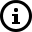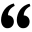# How to calculate the Depreciation on AssetsAdditional Deprecation is depend on Purchases assets  during  180 days or more Purchase Less than 180 days in a year,
If asset is put to use for less than 180 days then amount equal to 50% of the amount calculated using normal depreciating rates is allowed as depreciation.  Asset put to use on or before 5th oct of the year then 100% depreciation is allowed, otherwise 50%.let's understand this with following example in software.Here - Block of asset on Buildings  rate 10 %
Opening wdv - 100000
deprecation - 10000
in addition "180 days or more"     Asset put to use on or before 5th oct is 100000 (deprecation 10 % = 10000 )
in addition "less then 180 days"   Asset put to use on or after 5th oct is 100000 (deprecation 5 % = 5000 )

Here to total deprecation is 25000/=Hope this helps